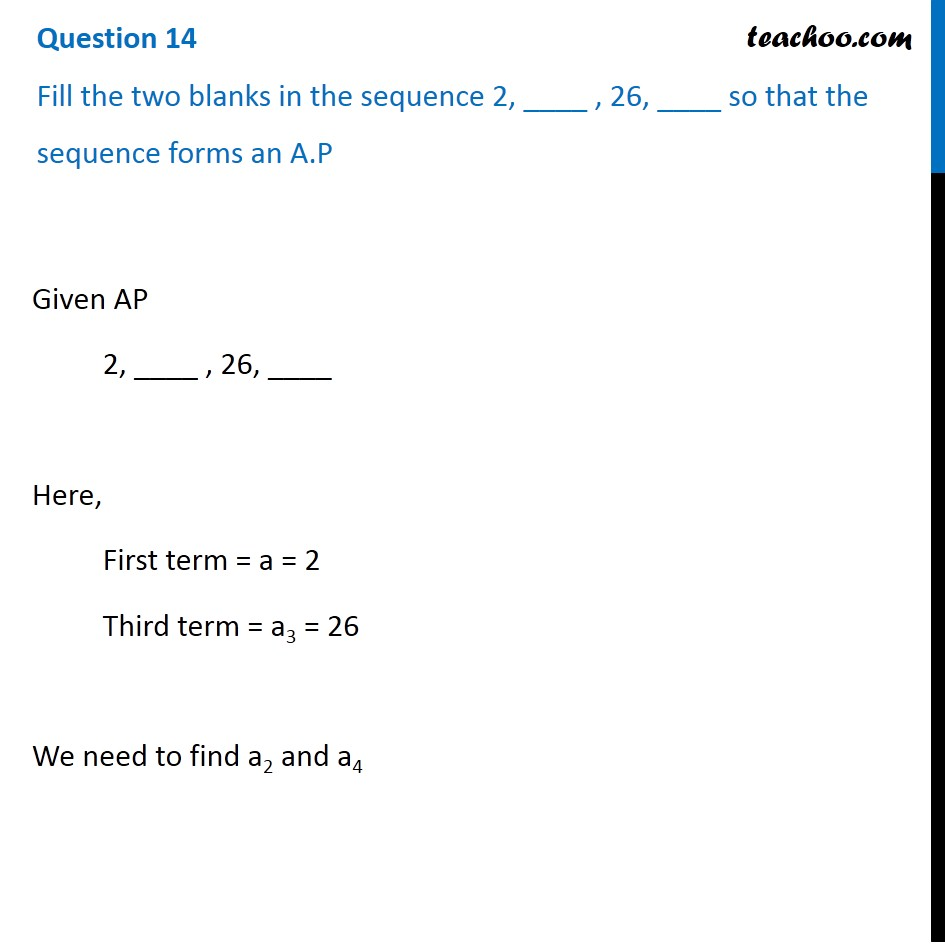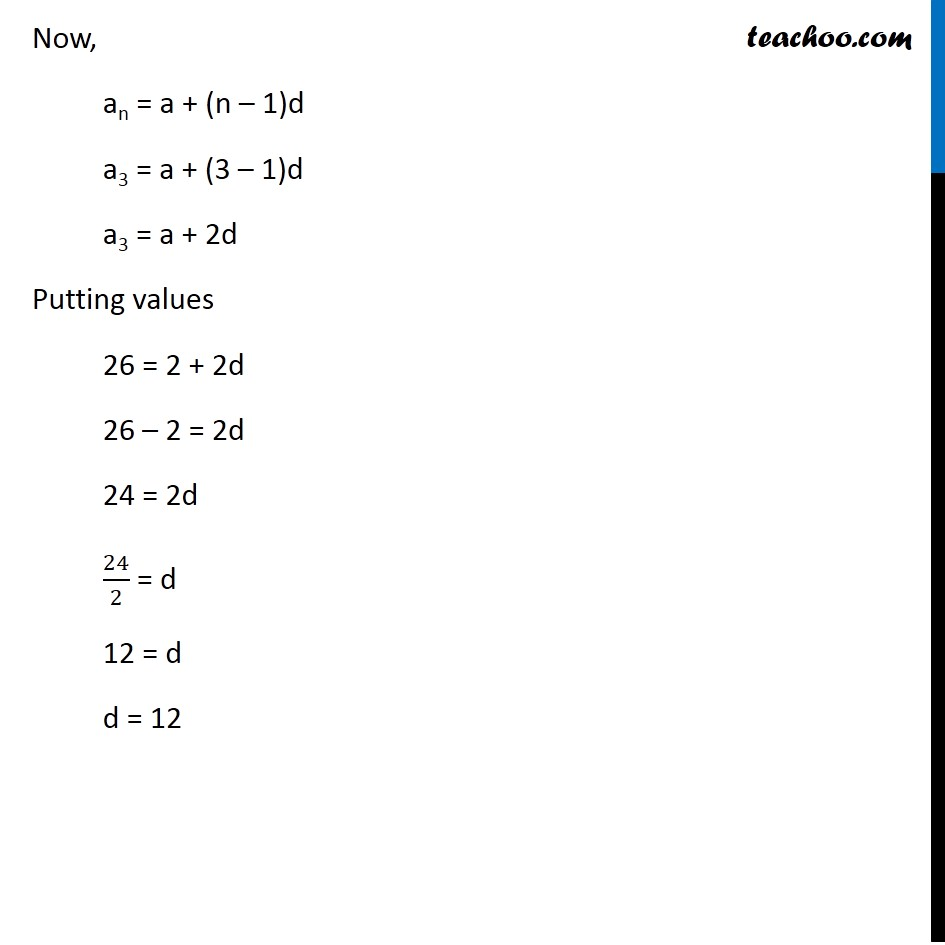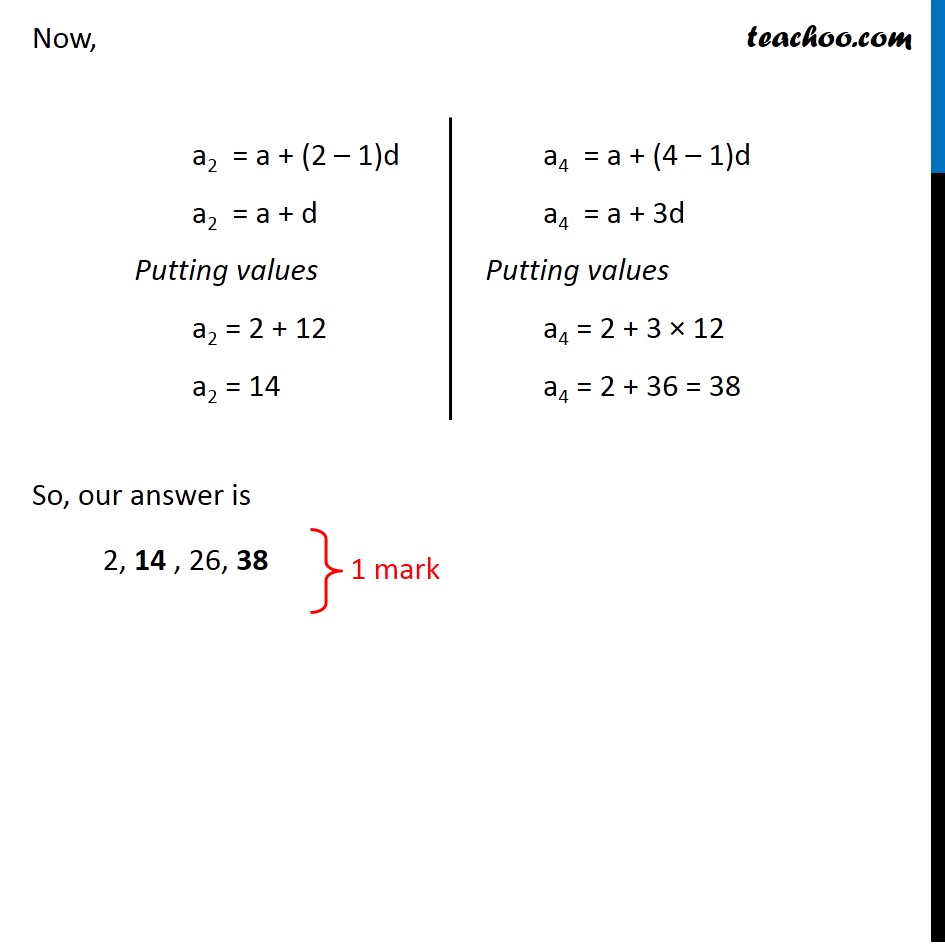CBSE Class 10 Sample Paper for 2020 Boards - Maths Standard

Class 10
Solutions of Sample Papers for Class 10 Boards

## Fill the two blanks in the sequence 2, ____ , 26, ____ so that the sequence forms an A.PNote : This is similar to Ex 5.2, 3 ( i ) of NCERT – Chapter 5 Class 10

Learn in your speed, with individual attention - Teachoo Maths 1-on-1 Class

### Transcript

Question 14 Fill the two blanks in the sequence 2, ____ , 26, ____ so that the sequence forms an A.P Given AP 2, ____ , 26, ____ Here, First term = a = 2 Third term = a3 = 26 We need to find a2 and a4 Now, an = a + (n – 1)d a3 = a + (3 – 1)d a3 = a + 2d Putting values 26 = 2 + 2d 26 – 2 = 2d 24 = 2d 24/2 = d 12 = d d = 12 Now, a2 = a + (2 – 1)d a2 = a + d Putting values a2 = 2 + 12 a2 = 14 a4 = a + (4 – 1)d a4 = a + 3d Putting values a4 = 2 + 3 × 12 a4 = 2 + 36 = 38 So, our answer is 2, 14 , 26, 38